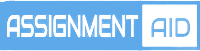# Regression analysis help#### Regression analysis help

##### REGRESSION HOMEWORK HELP

Regression analysis is a statistical method that allows you to examine the relationship between two or more variables of interest and finds great use in statistical prediction and forecasting. It helps determine the influence of one or more independent variables on a dependent variable thus determining what factor matters most or what factor can be ignored depending on the case scenario you may be dealing with. A dependent variable in this case is the main factor you are trying to understand or predict while the independent variables are the factors you have reason to believe have an impact on your dependent variable.  Therefore to conduct a regression analysis, you will need to first define your dependent variable that is being influenced by your independent variable then establish a dataset to work with. There are various types of regression analysis and they include linear regression, logistic regression and polynomial regression.

##### LINEAR REGRESSION HOMEWORK HELP

This is where the dependent variable is continuous whereas predictor variable(s) can be continuous and discrete. It establishes a relationship between the dependent variable (Y) and one or more predictor variables (x) using the best fit line whose nature is linear. Simple linear regression examines the relationship between one dependent variable and one predictor (independent) variable. If the model includes more than one predictor or independent variables, it is called multiple linear regression.

##### LOGISTIC REGRESSION HOMEWORK HELP

Logistic regression measures the relationship between categorical dependent variable and one of more predictor variables. The model estimates the probabilities using a logistic function which is cumulative logistic distribution.

##### RIDGE REGRESSION HOMEWORK HELP

Ridge Regression is a technique that is used when there is multicollinearity in the data involved.

##### LASSO REGRESSION HOMEWORK HELP

Researchers use Lasso Regression when high levels of accuracy are required. It also gives results with low variability.

##### HANDLING A REGRESSION ANALYSIS HOMEWORK HELP

To handle a regression analysis homework one needs to

1. Define your research question from which you choose your dependent and independent variables.
2. Following this, develop a hypothesis for the study and you are ready to conduct your analysis.

However, it is important to note that you have to ensure you meet all assumptions which include;

1. Linearity were all variables should be linear in nature.
2. Absence of multi-collinearity.
3. Normality where you need to ensure the data in question follows a normal distribution.
##### LOGISTIC REGRESSION HOMEWORK, SIMPLE LINEAR REGRESSION HOMEWORK, EXPONENTIAL REGRESSION HOMEWORK HELP

Regression deals with basic statistical concepts but can be complex at times. Whether in data collection, data analysis, results interpretation or data reporting, some students are likely to face a challenge thus need for online assignment or tutoring. This is why for your regression analysis homework help, we have a team of reliable experts offering you the help you may need in any but not limited of the following topics.

1. Simple Linear Regression
2. Confidence Intervals for Y
3. Non-parametric regression
4. The general Regression Situation
5. Common misconceptions about fit; multi-collinearity, regression modeling, fitted regression

## Example Samples

live:topwrit

###### Email:

support@assignmentsaid.com

###### Open Hours:

Mon-Sat: 9am - 6pm